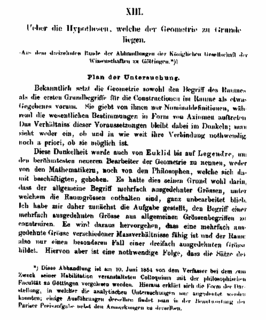## Saturday, August 27, 2005

### On the Hypothese at the foundations of GeometryI am trying to make my case on the greatest physics paper over at Cosmic Variance. One notices the slight misinterpretation I assigned, "geometical propensity to physics" that the case is more then just physi,s but the limmerack added envisioned, over such a paper that leads into physics.:) I see no difference now. So I refer to it as the greatest physics paper!

The title of this thread is attributed to Bernhard Riemann and a paper he wrote that revolutionized our concept of space with geometry of distances(the metric). With Gauss's tuteluge on curvature that was being developed, Reimann moved to understand how such changes now would be considered, where space is no longer flat. He moved Pythagorean thereom from:

c2=a2+b2 to c2=a2+b2-2ab cos Æ

where the right angle is no longer right but has magitude Æ then the above theorem has been generalized

The function that measures the instantaneous distance between two points was later used by Einstein where m and n vary over the intergers 1 and 2

ds2=gmndxmdxn

On the Hypothese at the foundations of GeometryBy use of similar triangles and congruent parts of similar triangles on the Saccheri quadrilateral, ABDC with AC = BD and ‚A = ‚B = p/2, he establishes his first 32 theorems. Most are too complicated to be treated in a short paper, but here some examples are merely stated, some are illustrated and some are proven. For those proofs which are brief enough to show here, the main steps are indicated and the reader is invited to fill in the missing details of the argument. A century after Saccheri, the geometers, Lobachevsky, Bolyai and Gauss would realize that, by substituting the acute case or the obtuse case for Euclid's postulate Number V, they could create two consistent geometries. In doing so they built on the progress made by Saccheri who had already proven so many of the needed theorems. They were able to create what we recognize today as the "elliptical" and "hyperbolic" non-Euclidean geometries. Most of Saccheri's first 32 theorems can be found in today's non-Euclidean textbooks. Saccheri's theorems are prefaced by "Sac."

How far advanced our thinking has become, that we can move quickly here to other avenues of consideration? How much "inbetween" the leading thinking of Riemann that we can have gotten here in our "physics of geometries?" Is it a suttle generalization in words and limmerack that such a physics view could have seen nature at its finest, and explained in a mathematical way.

Gaussian Coordinates
We can sum this up as follows: Gauss invented a method for the mathematical treatment of continua in general, in which ?size-relations? (?distances? between neighbouring points) are defined. To every point of a continuum are assigned as many numbers (Gaussian co-ordinates) as the continuum has dimensions. This is done in such a way, that only one meaning can be attached to the assignment, and that numbers (Gaussian co-ordinates) which differ by an indefinitely small amount are assigned to adjacent points. The Gaussian co-ordinate system is a logical generalisation of the Cartesian co-ordinate system. It is also applicable to non-Euclidean continua, but only when, with respect to the defined ?size? or ? distance,? small parts of the continuum under consideration behave more nearly like a Euclidean system, the smaller the part of the continuum under our notice.

Yes so easy now that we can see this space in ways that the average person without the physics comprehension would have never found that the fancy brane worlds held to perspective on the developing sciences and recognition of such physics processes had been elevated.

Would the likes of a Peter Woit be stagnated on what he sees if such limitation to the math endowed creator of mind, would see that such limitations to spintronic value added, would only partake of the events held to this brane and that a wider audience would now see that such dynamicsi n this universe would be greatly enhanced by entering a whole new world of abstraction.According to Einstein's general theory of relativity, the gravitational potential due to an isolated source is proportional to rho + 3P, where rho is the energy density and P is the pressure. For non-relativistic matter the pressure is negligibly small, whereas for radiation P = rho/3. Therefore, for the same value of the energy density, radiation produces a deeper and more attractive gravitational potential (left) than non-relativistic matter (centre). If rho + 3P is negative, as in the case of quintessence ­ in this example P = ­2rho/3 ­ the sign of the gravitational field is transformed from attractive to repulsive (right).